#### Chapter 3 Rationalization R.D. Sharma Solutions for Class 9th Math Exercise 3.2

Exercise 3.2

1. Rationalise the denominator of each of the following (i - vii) .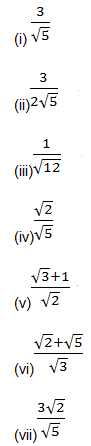Solution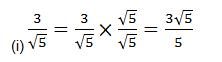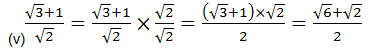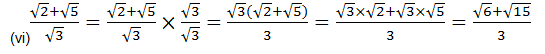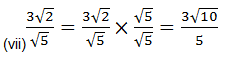2. Find the value o three places of decimals of each of the following It is given that Given 2 = 1.414, 3 = 1.732, 5 = 2.236 and 10 = 3.162.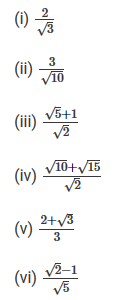Solution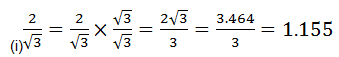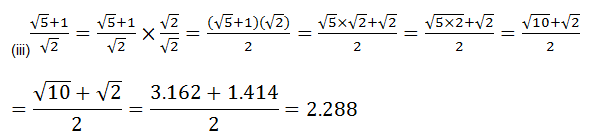(iv)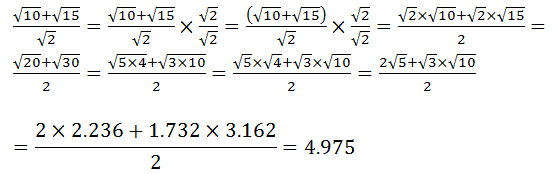3. Express each one of the following with rational denominator:Solution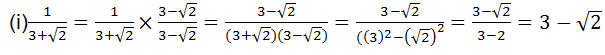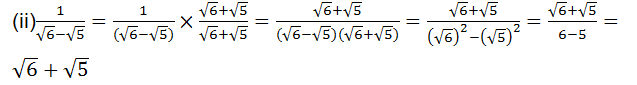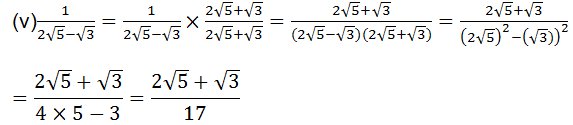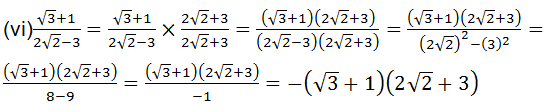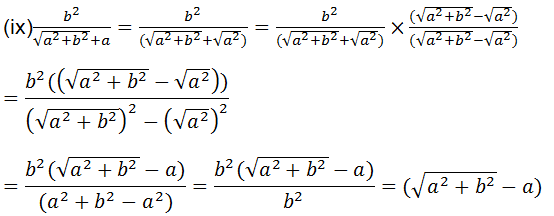4. Rationales the denominator and simplify :Solution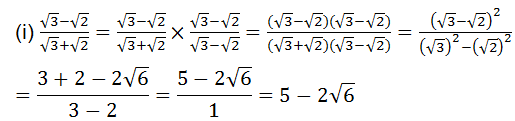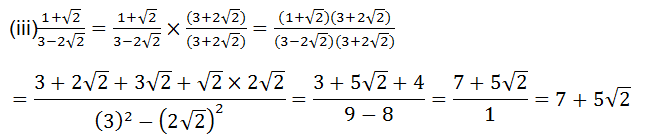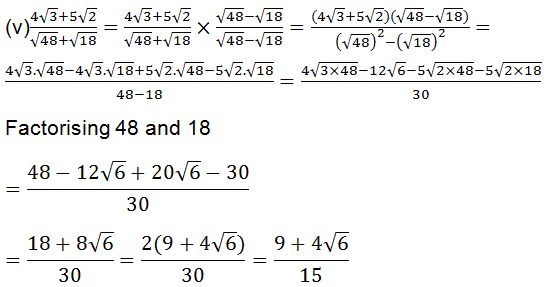5. Simplify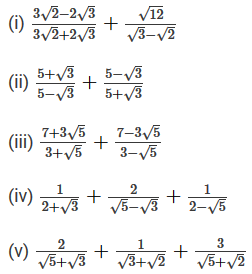Solution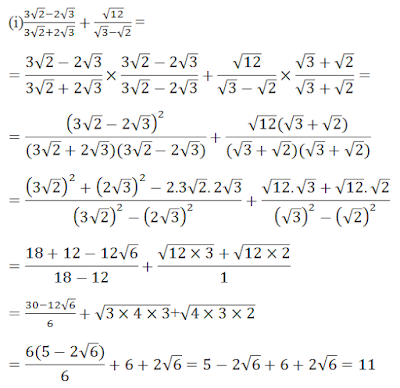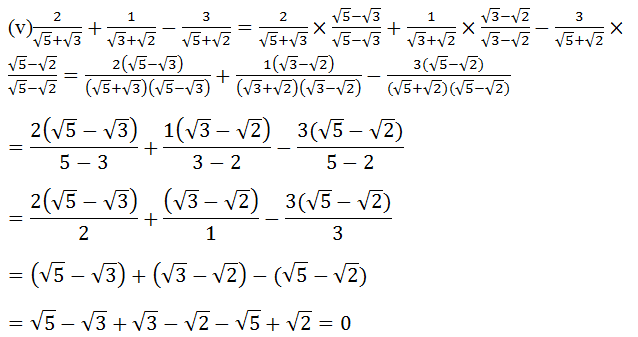6. In each of the following determine rational number a and b.Solution

(i)(vi)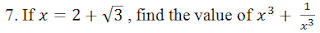Solution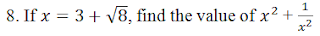Solution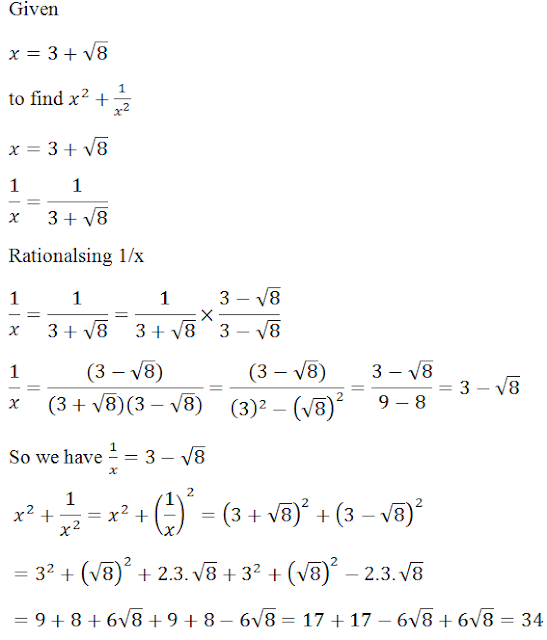Solution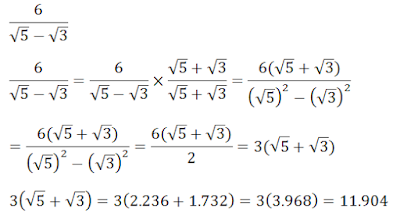10.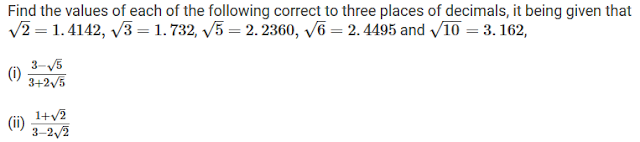Solution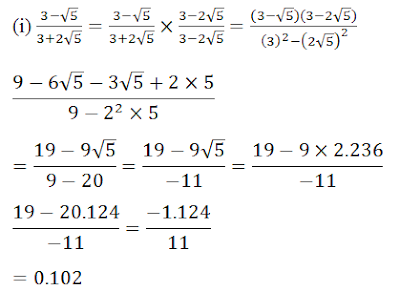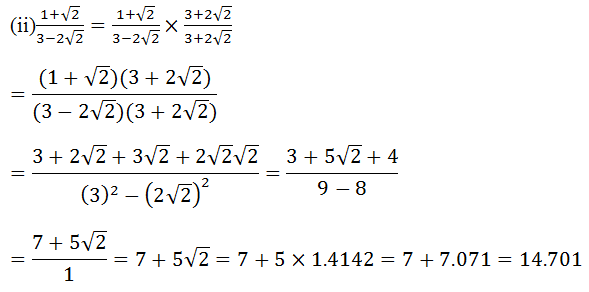Solution